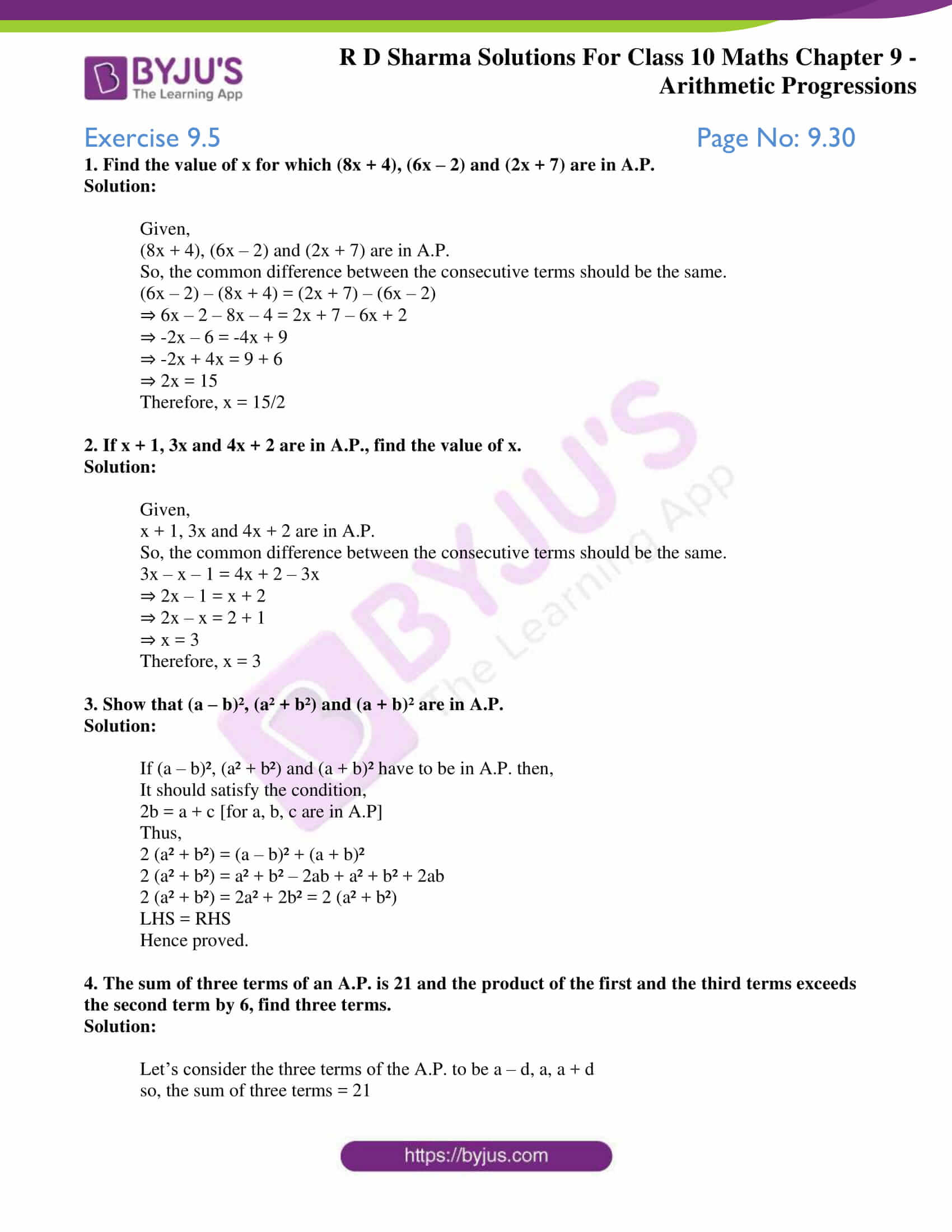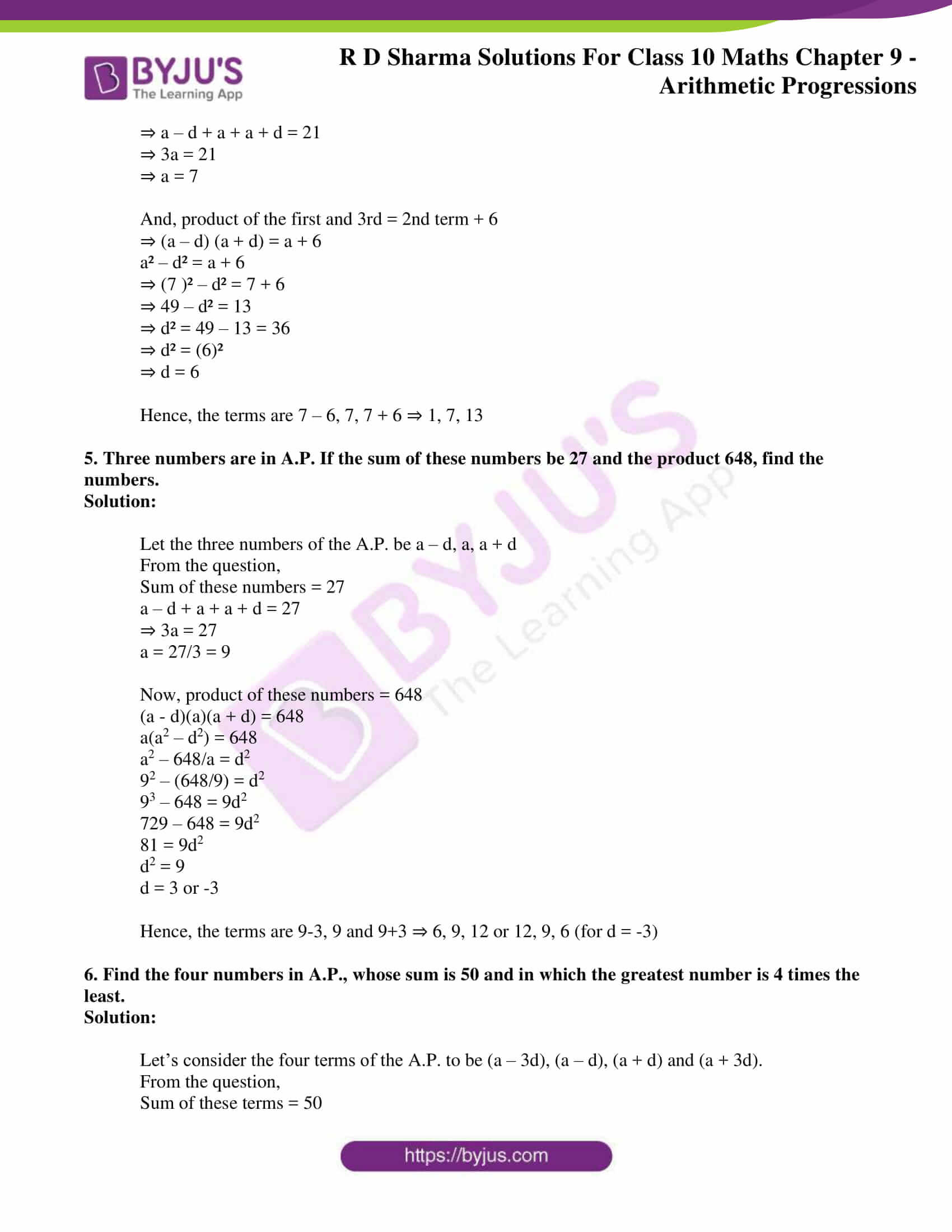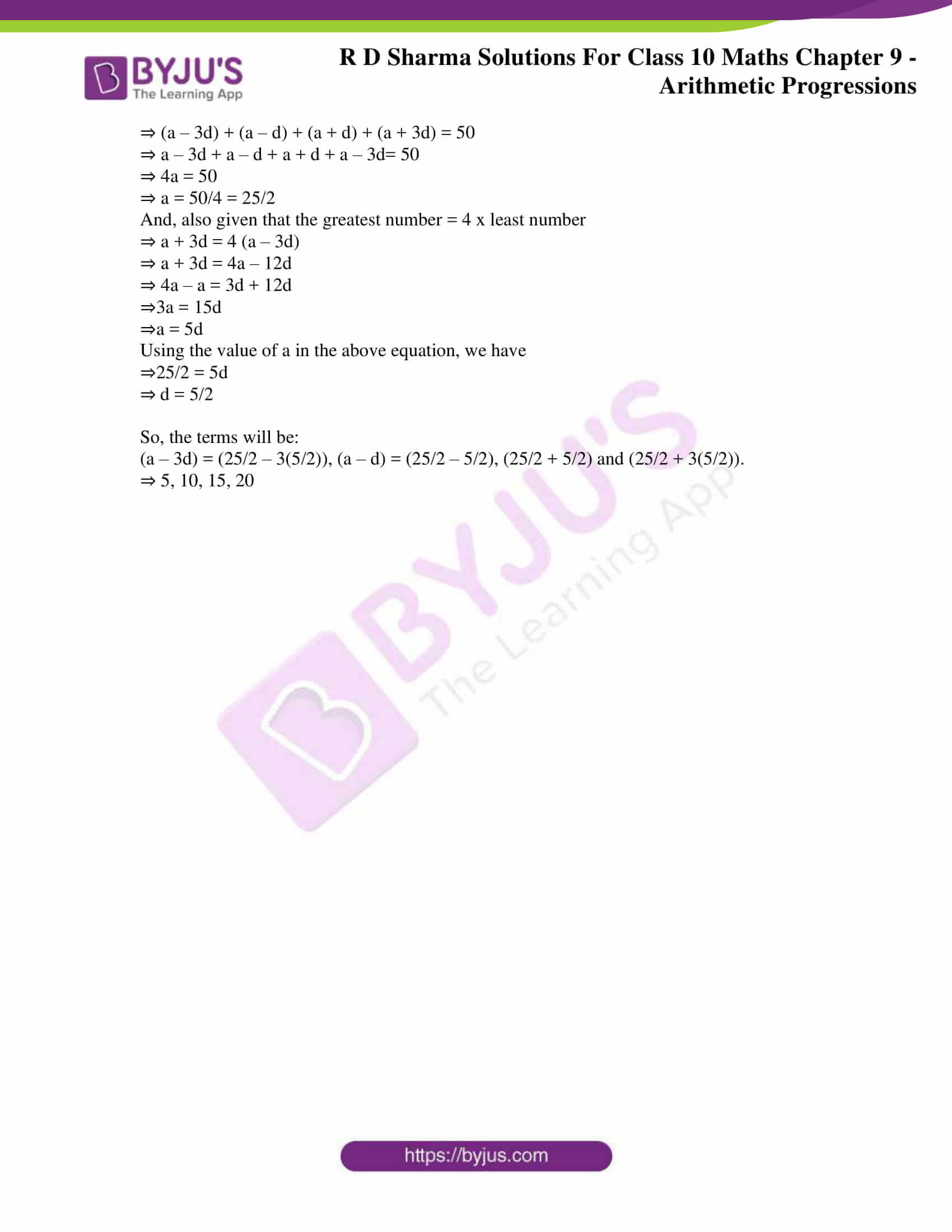# RD Sharma Solutions for Class 10 Maths Chapter 9 Arithmetic Progressions Exercise 9.5

The RD Sharma Solutions Class 10 chapter 9 exercise 9.5 majorly focuses on the selection of terms in an A.P. Several interesting problems in this exercise build upon a strong foundation over the main concept. BYJU’S has created solutions to these exercise problems with the main intention to clarify the doubts and strengthen the knowledge in A.P. Students can also download the RD Sharma Solutions for Class 10 Maths Chapter 9 Arithmetic Progressions Exercise 9.5 PDF is given below.

## RD Sharma Solutions for Class 10 Maths Chapter 9 Arithmetic Progressions Exercise 9.5 Download PDF### Access RD Sharma Solutions for Class 10 Maths Chapter 9 Arithmetic Progressions Exercise 9.5

1. Find the value of x for which (8x + 4), (6x – 2) and (2x + 7) are in A.P.
Solution:

Given,

(8x + 4), (6x – 2) and (2x + 7) are in A.P.

So, the common difference between the consecutive terms should be the same.

(6x – 2) – (8x + 4) = (2x + 7) – (6x – 2)

⇒ 6x – 2 – 8x – 4 = 2x + 7 – 6x + 2

⇒ -2x – 6 = -4x + 9

⇒ -2x + 4x = 9 + 6

⇒ 2x = 15

Therefore, x = 15/2

2. If x + 1, 3x and 4x + 2 are in A.P., find the value of x.
Solution:

Given,

x + 1, 3x and 4x + 2 are in A.P.

So, the common difference between the consecutive terms should be the same.

3x – x – 1 = 4x + 2 – 3x

⇒ 2x – 1 = x + 2

⇒ 2x – x = 2 + 1

⇒ x = 3

Therefore, x = 3

3. Show that (a – b)², (a² + b²) and (a + b)² are in A.P.
Solution:

If (a – b)², (a² + b²) and (a + b)² have to be in A.P. then,

It should satisfy the condition,

2b = a + c [for a, b, c are in A.P]

Thus,

2 (a² + b²) = (a – b)² + (a + b)²

2 (a² + b²) = a² + b² – 2ab + a² + b² + 2ab

2 (a² + b²) = 2a² + 2b² = 2 (a² + b²)

LHS = RHS

Hence proved.

4. The sum of three terms of an A.P. is 21 and the product of the first and the third terms exceeds the second term by 6, find three terms.
Solution:

Let’s consider the three terms of the A.P. to be a – d, a, a + d

so, the sum of three terms = 21

⇒ a – d + a + a + d = 21

⇒ 3a = 21

⇒ a = 7

And, product of the first and 3rd = 2nd term + 6

⇒ (a – d) (a + d) = a + 6

a² – d² = a + 6

⇒ (7 )² – d² = 7 + 6

⇒ 49 – d² = 13

⇒ d² = 49 – 13 = 36

⇒ d² = (6)²

⇒ d = 6

Hence, the terms are 7 – 6, 7, 7 + 6 ⇒ 1, 7, 13

5. Three numbers are in A.P. If the sum of these numbers be 27 and the product 648, find the numbers.
Solution:

Let the three numbers of the A.P. be a – d, a, a + d

From the question,

Sum of these numbers = 27

a – d + a + a + d = 27

⇒ 3a = 27

a = 27/3 = 9

Now, product of these numbers = 648

(a – d)(a)(a + d) = 648

a(a2 – d2) = 648

a2 – 648/a = d2

92 – (648/9) = d2

93 – 648 = 9d2

729 – 648 = 9d2

81 = 9d2

d2 = 9

d = 3 or -3

Hence, the terms are 9-3, 9 and 9+3 ⇒ 6, 9, 12 or 12, 9, 6 (for d = -3)

6. Find the four numbers in A.P., whose sum is 50 and in which the greatest number is 4 times the least.
Solution:

Let’s consider the four terms of the A.P. to be (a – 3d), (a – d), (a + d) and (a + 3d).

From the question,

Sum of these terms = 50

⇒ (a – 3d) + (a – d) + (a + d) + (a + 3d) = 50

⇒ a – 3d + a – d + a + d + a – 3d= 50

⇒ 4a = 50

⇒ a = 50/4 = 25/2

And, also given that the greatest number = 4 x least number

⇒ a + 3d = 4 (a – 3d)

⇒ a + 3d = 4a – 12d

⇒ 4a – a = 3d + 12d

⇒3a = 15d

⇒a = 5d

Using the value of a in the above equation, we have

⇒25/2 = 5d

⇒ d = 5/2

So, the terms will be:

(a – 3d) = (25/2 – 3(5/2)), (a – d) = (25/2 – 5/2), (25/2 + 5/2) and (25/2 + 3(5/2)).

⇒ 5, 10, 15, 20